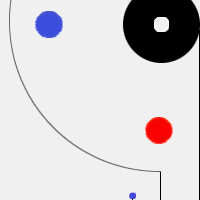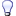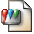Home » Audio » Group Build » CSD01060 DIODE If / Uf and If / rD CURVES (Measuring, calculating, drawing, sharing.)
CSD01060 DIODE If / Uf and If / rD CURVES Thu, 05 April 2018 13:46FL152 Messages: 29Registered: April 2011 Chancellor
Forward Voltage Uf vs. Forward Current If values of one (randomly selected) CSD01060 HV SiC Schottky diode are measured with regulated lab DC supply and quality (Fluke 87V) multimeter. The aim was determining of suitability for tube cathode bias (instead of Rk // Ck „standard" solution). Two things are of interest here:

1.) Values of Uf vs. changing If for low currents, typical for many tubes (say 1-70 mA)
2.) Dynamic resistance rD (for AC) values for various If . We need really low numbers here, because anode resistance of triode will rise for ( μ + 1 ) x rD from ideal, Rk = 0 (Rk bypassed with Ck for AC) state. Especially when we`d need 2-3-4 (or even more) diodes in series, because of relative low Uf per diode.

For example, trioded D3a (μ = 70) with bypassed Rk has anode resistance of about 3 kΩ with Ia = 10-11 mA. Biased with two CSD01060 in series (Uk = 2 x Uf = 1,74 V), anode resistance is now larger, ra ~ 3,5 kΩ because of (unbypassed for AC) rD = 6,5 Ω in cathode path. If (and how much) it matters depends of implementation. One example where it is critical is RIAA stage.Figure 1: Forward current vs. Forward Voltage Curve for CSD01060, SiC Schottky diode

Determining of Dynamic Resistance rD at a certain forward current, example 10 mA:

- At If = 10 mA (reads Uf = 0,87 V) point on If / Uf graph draw the tangent line

- Calculate rD = (ΔU_F)/(ΔI_F ) = (0,95-0,84)/(0,034-0) = 3,24 OhmsFigure 2: Graphic determining of Dynamic Resistance rD - Tangent line to the If / Uf slope

Several rD values vs. If gives a new graph, where we can see how rD shifts with changing If . It is obvious that diode is quite capable of acceptable low rD when we are „away" from the „knee", about ≥ 10 mA.

Another example: Eleven diodes in series would be suitable for (cathode) bias PP EL84 output stage. About 58,5 mA DC standing current, 11 x 0,934 V = 10,27 V, where rD would be 11 x 0,5 = 5,5 Ohms. Large current „reserve" (2 A for CSD1060) is a another „bonus" on current peaks.Figure 3: Dynamic Resistance rD vs. forward current If curve of CSD01060 diode

•Attachment: graf1.tif
•Attachment: graf2.tif
•Attachment: graf3.tif